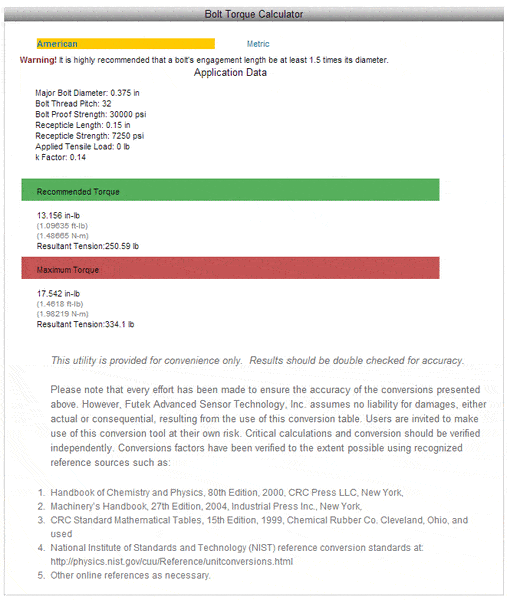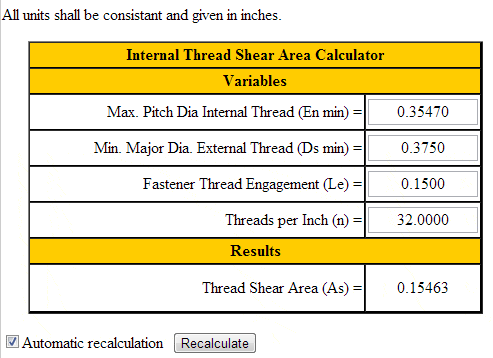# Screw and mating thread strength equations

I am designing a mating male and female threaded interface, where the nut is much weaker than the male thread. I need to be able to calculate a few things:
A. The maximum force (as preload) required to reach the yield point of the nut material.
B. The torque required to achieve the above.

The thread is 3/8-32 UNEF. The male part is either nickel plated brass or zinc, and the female part is injection moulded ABS.
The length of engagement is 0.15".

I have found a few equations, but different sources list different methods. What I have also found is nobody cares how much torque it requires to strip a thread, as the more common goal is to calculate how much preload is required to yield the shank.

First, I put the numbers into the FUTEK bolt torque calculator:17in-lb sounds like a reasonable figure for maximum torque and near what I'd expect.

It would be nice if FUTEK displayed the equations they derived their answers from, but they don't.

So, here are some of the methods and equations I have found:

1. ATS = ∏nLeDsmin((1/2n) + 0.57735(Dsmin - Emax))

Where:
ATS = Shear area of root of nut threads (in2)
n = threads per inch = 32
Le = length of thread engagement = 0.15"
Dsmin = minimum OD of bolt threads = 0.375"
Enmax = maximum PD of nut = 0.3547"*

*Calculated using:
Enmax = dp = D - 0.64592p
Where p = 1/32
I confirmed the pitch diameter of 0.3547" from this chart.

From 1:
ATS = 0.1546 if n=32, 0.0884 if n=1/32.

Another equation I found for calculating thread shear area is:

2. Ath = 0.5∏dpLe

Where:
dp = pitch diameter of thread = 0.3547"
Le = length of thread engagement = 0.15"

From 2:
Ath = 0.0836. This isn't far off of the second answer from 1.

A third option from Engineer's Edge is an online calculator:This matches the first answer of 0.1546in2 from 1.

OK, if I take those two numbers and put them into:

3. F = SUATS

Where:
SU = ultimate shear strength of the nut or bolt materials. In this case, the nut is the weaker material. The nuts is to be made of ABS, SU = 3625psi, taken from tensile strength * 0.5.
ATS = 0.1546in2 and 0.0884in2 taken from 1.

∴ if ATS = 0.1546, then F = 560.425lb
if ATS = 0.0884, then F = 320.45lb

Using this, then calculate torque required using:

4. Tin = F((P/2∏) + ((μtrt)/cosβ) + μnrn)

Where:
Tin = torque applied to the fastener
F = preload created in the fastener
P = thread pitch in inches = 1/32
μt = the coefficient of friction between the nut and bolt threads = 0.15
rt = the effective contact radius of the threads = 0.3547 = dp (I am unsure of this being correct)
β = half of the angle of the thread = 30°
μn = the coefficient of friction between the face of the nut and the upper surface of the joint (not relevant) = 0
rn = the effective radius of contact between the nut and joint surface (not relevant) = 0

But there is another equation, and completely different method, here.
I believe this link is calculating when the shank will fail, as the equation for F uses σT as opposed to SU.

Using 4:
If F = 560.425lb, Tin = 37.21in-lb
If F = 320.45lb, Tin = 21.28in-lb

The second number, 21.28in-lb seems to be within the range of what the FUTEK bolt torque calculator suggested. However, there are so many variables I am not certain of within these equations that I need to know more and if I am going wrong somewhere.

Please can anyone offer some advice, if not a definitive answer?

Many thanks.

Last edited:

## Answers and Replies

In addition to the friction between the mating threads (which you accounted for), you need to account for the friction between the head of the bolt and whatever surface it is coming in contact with.

Ah, yes. The nut doesn't come into contact with a mating face, instead the mating faces are stationary inside the nut using a slip-ring mechanism. Thanks, I must take the friction and effective radius into account.

Is effective radius the mean diameter of the contact surfaces?

Thanks.

I'll try to give some input from stress principals.
First of all I'm a South African so imperial units confuse the hell out of me, but that is a discussion for another day, haha...

Obviously you did some homework...
I'm gonna use a M10 TR GR8.8 bolt say with a S30400 nut... with the nut being weaker...
Nut material: BS 970 PART 4 GR 304 S15. It is assumed that the following properties apply to the material:
Minimum ultimate tensile strength: Sut = 505 MPa
Tensile yield strength: Syt = 205 MPa
Shear ultimate strength: Ssu = 0.6x505 MPa = 303 MPa
Shear yield strength: Ssy = 0.577x205 MPa = 118 MPa
Modulus of elasticity: E =193 GPa

Nut thickness: a = 8 mm
Depth of thread: t = 1 mm
Pitch: p = 2 mm
Thread height for TR thread: h = 0.634*p = 1.268 mm
Number of threads taking load: It is assumed that only 2 threads take the load.
Thread length: b = ∏*d_m*n = ∏(9.25)(2) = 58.12 mm

Stresses acting on the thread:
Use weaker thread as design criteria:
Please note that yielding will first take place but if you want to know the force at the point where the thread will rupture use rupture tensile strength:
Bending: σ_bending=My⁄I
with M = Ft/2; y = h/2 and I = bh^3/12
Thus σ_bending=3Ft⁄(bh^2 )
505=3F(1)⁄((58.12*1.268^2 )
F = 15.73 kN
Shear: tau_max=1.5F⁄bh
303=1.5F⁄(58.12*1.268)
F = 14.89 kN
Because bending is involved, the shear stress distribution is not uniform; it occurs at the centre of the thread and is 50% higher than the average stress. At this point the bending stress is zero because maximum bending stress occurs at the outer fibres. And this stresses will not be combined:

Hence rupture will occur due to shear at 14.89 kN and this translates to roughly 29 Nm, didn't do the exact calculations here.

Note this is only for the threads, you can do some calcs on the bolt aswell but seeing that the material of the bolt is stronger it can be thought logical that it will not break before the nut threads...

Remember that if you want safe working load than use the yielding stresses and check the resulting thrusts and torques.

Hoped this helped a bit, not an expert, just the type of calculations I would do for this problem, so just use your parameters. Hope I did not give you the direct answer as I will get some stick from some of the PF police guys, haha...

I'm English and was born after metrication so pounds are quite alien to me too. I assumed this forum was largely American hence the unit choice. And the thread is in inches, so it makes sense to use pounds.

Thanks for your reply - I am now confused.
Are you assuming two turns of the cross-section of the thread are effectively a beam with a uniformly distributed load? I don't understand how it is that simple, as the thread is attached to the nut...
Or is it a cantilever beam, 58.12mm long with a 1.268mm overhang, trapezoidal in section?

I also don't understand how you got this:
Thus σ_bending=3Ft⁄(bh^2 )
505=3F(1)⁄((58.12*1.268^2 )

From this:
σ_bending=My⁄I
with M = Ft/2; y = h/2 and I = bh^3/12

I try putting numbers back into My/I and get very different answers...

Thanks.

mc172, yes you treat it as a cantilever attached to the nut itself. Yes the threads will almost definitely not distribute the entire load evenly between them, mostly the firt two threads of your nut take the entire load, this is what I am assuming in my calcs.
Remember this will be the peak bending stress in the thread.
"σ_bending=My⁄I
with M = Ft/2; y = h/2 and I = bh^3/12"

By substituting we get σ_bending = (Ft/2)*(h/2)/bh^3/12
So σ_bending =(Fth/4)*(12/bh^3) hence σ_bending =3Ft⁄(bh^2) Hope you see this?